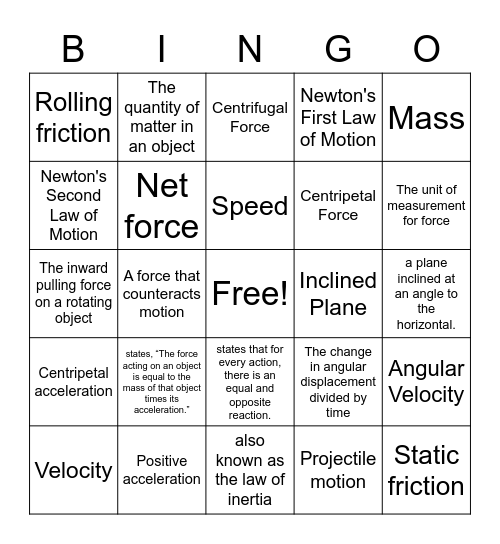# PHYSICS BINGOThis bingo card has a free space and 60 words: Acceleration, Positive acceleration, Negative acceleration, Centripetal acceleration, Projectile motion, Force, Net force, Equilibrium, Centripetal Force, Newton, Newton's First Law of Motion, Inertia, Newton's Second Law of Motion, Newton's Third Law of Motion, Friction, Rolling friction, Static friction, Mass, Weight, Speed, Inclined Plane, Centrifugal Force, Velocity, Angular Velocity, A push or a pull, when the net force on an object is zero, An object at rest remains at rest; and if in motion will remain in motion in a straight line; unless a force acts on it, This acts on a mass to create the force of weight, The unit of measurement for force, The sum of all forces acting on an object, An objects resistance to change its motion, The rate at which velocity is changing, Magnitude of distance traveled divided by time taken, speed + direction, The quantity of matter in an object, The pull of gravity on an object's mass (mg=), An objects resistance to change in its motion, A force that counteracts motion, The apparent outward force acting on a rotating abject, The inward pulling force on a rotating object, Force that is always present & keeps things upright, states, “The force acting on an object is equal to the mass of that object times its acceleration.”, a plane inclined at an angle to the horizontal., The unit of measurement for forces, The change in angular displacement divided by time, A negative change in velocity, A positive change in velocity, the resistance force that slows down the motion of a rolling ball or wheel., the frictional force that prevents two surfaces from sliding past each other, states that for every action, there is an equal and opposite reaction., also known as the law of inertia, p=mv, velocity at a specific time 't', Rise over run, the gravitationally curved path of an object around a point in space, combination of the standard three additive primary colors, each of the digits of a number that are used to express it to the required degree of accuracy, starting from the first nonzero digit., a change in position of an object, states that the force (F) needed to extend or compress a spring by some distance X is proportional to that distance. and the property of matter that measures its resistance to acceleration.

## Play Online